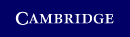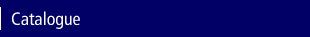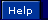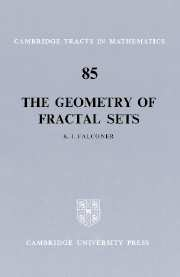Home > Catalogue > The Geometry of Fractal SetsSearch this book

## Details

• Page extent: 180 pages
• Size: 228 x 152 mm
• Weight: 0.27 kg

### Paperback

(ISBN-13: 9780521337052 | ISBN-10: 0521337054)

• There was also a Hardback of this title but it is no longer available | Adobe eBook
• ### For price and ordering options, inspection copy requests, and reading lists please select:

Please note local prices may apply

This book contains a rigorous mathematical treatment of the geometrical aspects of sets of both integral and fractional Hausdorff dimension. Questions of local density and the existence of tangents of such sets are studied, as well as the dimensional properties of their projections in various directions. In the case of sets of integral dimension the dramatic differences between regular 'curve-like' sets and irregular 'dust like' sets are exhibited. The theory is related by duality to Kayeka sets (sets of zero area containing lines in every direction). The final chapter includes diverse examples of sets to which the general theory is applicable: discussions of curves of fractional dimension, self-similar sets, strange attractors, and examples from number theory, convexity and so on. There is an emphasis on the basic tools of the subject such as the Vitali covering lemma, net measures and Fourier transform methods.

### Contents

Preface; Introduction; Notation; 1. Measure and dimension; 2. Basic density properties; 3. Structure of sets of integral dimension; 4. Structure of sets of non-integral dimension; 5. Comparable net measures; 6. Projection properties; 7. Besicovitch and Kakeya sets; 8. Miscellaneous examples of fractal sets; References; Index.

### Review

'This book is filled with the geometric jewels of fractional and integral Hausdorff dimension. It contains a much-needed unified notation and includes many recent results with simplified proofs. The theory is classically and rigorously presented with applications only alluded to in the introduction. Each chapter contains a short and important problem set. This is a lovely introduction to the mathematics of fractal sets for the pure mathematician.' American Mathematical Monthly